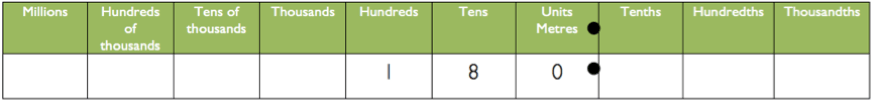# TEACH: Can I see an example of some planning for a numeracy activity?Here’s a different scenario and example of some planning for an activity that embeds some numeracy work into a foundation learning course with a focus on building and construction.

First, read through the scenario, learning outcome, and resources. Then have a look at the activity planned at the end.

# Scenario

You teach an entry-level Building and Construction course. Your learners need to work with metric measurement including for lengths of timber. But you also want them to learn to estimate quantities without always relying on the calculator on their phones.

# Learning outcome

Based on your mapping, diagnostic assessment and work with these learners you decided on the following as your intended learning outcome for your first attempt at embedding some numeracy into your content:

• Use strategies (including using a place value chart) to estimate and solve multiplication problems that require multiplying or dividing by 10, 100 and 1000.

# Activity

You’ve decided to walk them through some examples of how the maths works. You want them to do this mentally, but you decide to use a place value chart as a way of making it explicit to start with.

You will supply them with an example where they have to estimate and then calculate how much timber is needed.

From there, you can get the group to discuss their answers and you can go through and model the process using the place value chart.

After that, the learners can work in pairs and come up with examples for others to estimate and calculate using the same strategy.

# Resources

Here are the resources that you know you need:

• Blank place value charts that you can print out or photocopy.

# Planning

Here’s what your actual planning might look like for the guided teaching and learning sequence:

## Activity 1: Using a place value chart to estimate timber quantity

1. Draw up a place value chart on the whiteboard.
2. Provide the example: “Let’s say that you need to find out the following: What is the total amount of timber needed for 220 lengths of 87.5m?
3. Ask the learners to have a guess first, and write this down on a piece of paper.
4. Compare estimations from around the room with feedback to the board to show the range of answers.
5. Introduce the place value chart and model the estimation as follows: “Let’s simplify things… “220 lengths of 87.5 is roughly 200 lengths of 90m.”
6. Say the revised calculation: “We can calculate this quickly as two lengths of 90m times 100.”
7. Do the first part of the calculation then put this on the chart: “Two lengths of 90m is 180m.”
• Then to find 100 lengths of 180, shift the 180 two places to the left on the place value chart. This is 18 000m8. Extension:
• Provide other examples. Ask learners to work out the answers in any way they like but without using a calculator at this stage.
• Compare answers and try working it out on a calculator.## Author: Graeme Smith

Education, technology, design. Also making cool stuff...